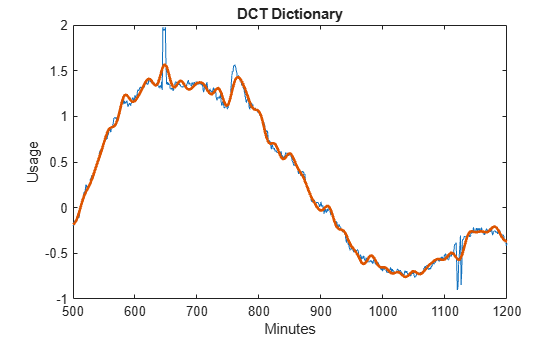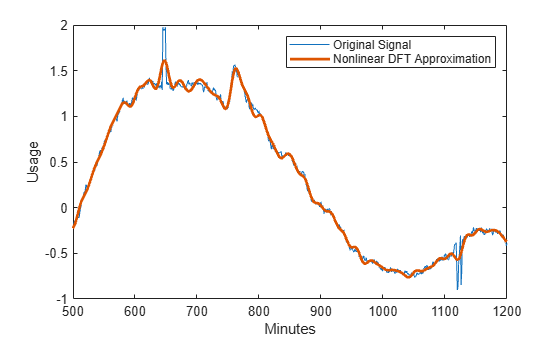## Matching Pursuit

### Matching Pursuit Dictionary Creation and Visualization

This example shows how to create and visualize a dictionary consisting of a Haar wavelet down to level 2.

```[mpdict,~,~,longs] = wmpdictionary(100,'lstcpt',{{'haar',2}}); ```

Use the `longs` output argument to divide the wavelet dictionary by level and type of function (scaling or wavelet). Step through a plot of the translated scaling functions and wavelets by level.

```for nn = 1:size(mpdict,2) if (nn <= longs{1}(1)) plot(mpdict(:,nn),'k','linewidth',2) grid on xlabel('Translation') title('Haar Scaling Function - Level 2') elseif (nn>longs{1}(1) && nn<= longs{1}(1)+longs{1}(2)) plot(mpdict(:,nn),'r','linewidth',2) grid on xlabel('Translation') title('Haar Wavelet - Level 2') else plot(mpdict(:,nn),'b','linewidth',2) grid on xlabel('Translation') title('Haar Wavelet - Level 1') end pause(0.2) end ```This animation infinitely loops through all the plots generated.### Orthogonal Matching Pursuit on a 1-D Signal

This example shows how to perform orthogonal matching pursuit on a 1-D input signal that contains a cusp.

Load the cuspamax signal. Construct a dictionary consisting of Daubechies least asymmetric wavelet packets at level 4, Daubechies extremal phase wavelets at level 2, the DCT-II basis, the sin basis, and the shifted Kronecker delta basis.

```load cuspamax; dict = {{'wpsym4',1},{'db4',2},'dct','sin','RnIdent'}; mpdict = wmpdictionary(length(cuspamax),'lstcpt',dict); ```

Use orthogonal matching pursuit to obtain an approximation of the signal in the overcomplete dictionary, mpdict, with 25 iterations. Plot the result as a movie, updating every 5 iterations.

```[yfit,r,coeff,iopt,qual] = wmpalg('OMP',cuspamax,mpdict,'typeplot',... 'movie','stepplot',5); ```### Electricity Consumption Analysis Using Matching Pursuit

This example shows how to compare matching pursuit with a nonlinear approximation in the discrete Fourier transform basis. The data is electricity consumption data collected over a 24-hour period. The example demonstrates that by selecting atoms from a dictionary, matching pursuit is often able to approximate a vector more efficiently with M vectors than any single basis.

Matching Pursuit Using DCT, Sine, and Wavelet Dictionaries

Load the dataset and plot the data. The dataset contains 35 days of electric consumption. Choose day 32 for further analysis. The data is centered and scaled, so the actual units of usage are not relevant.

```load elec35_nor x = signals(32,:); plot(x) xlabel('Minutes') ylabel('Usage')```The electricity consumption data contains smooth oscillations punctuated by abrupt increases and decreases in usage.

Zoom in on a time interval from 500 minutes to 1200 minutes.

`xlim([500 1200])`You can see the abrupt changes in the slowly-varying signal at approximately 650, 760, and 1120 minutes. In many real-world signals like these data, the interesting and important information is contained in the transients. It is important to model these transient phenomena.

Construct a signal approximation using 35 vectors chosen from a dictionary with orthogonal matching pursuit (OMP). The dictionary consists of the Daubechies extremal phase wavelet and scaling vectors at level 2, the discrete cosine transform (DCT) basis, a sine basis, the Kronecker delta basis, and the Daubechies least asymmetric phase wavelet and scaling vectors with 4 vanishing moments at levels 1 and 4. Then, use OMP to find the best 35-term greedy approximation of the electric consumption data. Plot the result.

```dictionary = {{'db1',2},{'db1',3},'dct','sin','RnIdent',{'sym4',4}}; [mpdict,nbvect] = wmpdictionary(length(x),'lstcpt',dictionary); [y,~,~,iopt] = wmpalg('OMP',x,mpdict); plot(x) hold on plot(y) hold off xlabel('Minutes') ylabel('Usage') legend('Original Signal','OMP')```You can see that with 35 coefficients, orthogonal matching pursuit approximates both the smoothly-oscillating part of the signal and the abrupt changes in electricity usage.

Determine how many vectors the OMP algorithm selected from each of the subdictionaries.

```basez = cumsum(nbvect); k = 1; for nn = 1:length(basez) if (nn == 1) basezind{nn} = 1:basez(nn); else basezind{nn} = basez(nn-1)+1:basez(nn); end end dictvectors = cellfun(@(x)intersect(iopt,x),basezind, ... 'UniformOutput',false);```

The majority (60%) of the vectors come from the DCT and sine basis. Given the overall slowly-varying nature of the electricity consumption data, this is expected behavior. The additional 14 vectors from the wavelet subdictionaries captures the abrupt signal changes. The number of vectors of each type is:

• 3 Daubechies wavelet (db4) level 2 vectors

• 16 Discrete cosine transform vectors

• 5 Sine vectors

• 2 Daubechies least-asymmetric wavelet (sym4) level 1 vectors

• 9 Daubechies least-asymmetric wavelet (sym4) level 4 vectors

Matching Pursuit Using DCT and Sine Dictionary vs. Full Dictionary

Repeat the OMP with only the DCT and sine subdictionaries. Set the OMP to select the 35 best vectors from the DCT-sine dictionary. Construct the dictionary and perform the OMP. Compare the OMP with the DCT-sine dictionary to the OMP with the additional wavelet subdictionaries. Notice that adding the wavelet subdictionaries shows the abrupt changes in electricity usage more accurately. The advantage of including the wavelet bases is especially clear especially in approximating the upward and downward spikes in usage at approximately 650 and 1120 minutes.

```dictionary2 = {'dct','sin'}; [mpdict2,nbvect2] = wmpdictionary(length(x),'lstcpt',dictionary2); y2 = wmpalg('OMP',x,mpdict2,'itermax',35); plot(x) hold on plot(y2,'linewidth',2) hold off title('DCT and Sine Dictionary') xlabel('Minutes') ylabel('Usage') xlim([500 1200])``````figure plot(x) hold on plot(y,'linewidth',2) hold off title('Full Dictionary') xlabel('Minutes') ylabel('Usage') xlim([500 1200])```Obtain the best 35-term nonlinear approximation of the signal in the discrete Fourier basis. Obtain the DFT of the data, sort the DFT coefficients, and select the 35 largest coefficients. The DFT of a real-valued signal is conjugate symmetric, so only consider frequencies from 0 (DC) to the Nyquist (1/2 cycles/minute).

```xdft = fft(x); [~,I] = sort(xdft(1:length(x)/2+1),'descend'); ind = I(1:35);```

Examine the vector `ind`. None of the indices correspond to 0 or the Nyquist. Add the corresponding complex conjugate to obtain the nonlinear approximation in the DFT basis. Plot the approximation and the original signal.

```indconj = length(xdft)-ind+2; ind = [ind indconj]; xdftapp = zeros(size(xdft)); xdftapp(ind) = xdft(ind); xrec = ifft(xdftapp); plot(x) hold on plot(xrec,'LineWidth',2) hold off xlabel('Minutes') ylabel('Usage') legend('Original Signal','Nonlinear DFT Approximation')```Similar to the DCT-sine dictionary, the nonlinear DFT approximation performs well at matching the smooth oscillations in electricity consumption data. However, the nonlinear DFT approximation does not approximate the abrupt changes accurately. Zoom in on the interval of the data containing the abrupt changes in consumption.

```plot(x) hold on plot(xrec,'LineWidth',2) hold off xlabel('Minutes') ylabel('Usage') legend('Original Signal','Nonlinear DFT Approximation') xlim([500 1200])```## SupportGet trial now